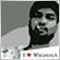# EA Shift to Pip Error ?470

```//+------------------------------------------------------------------+
//| Expert initialization function                                   |
//+------------------------------------------------------------------+
int OnInit()
{
magic=MagicNumberGenerator();
Print("MagicNumber is ",magic);
HideTestIndicators(false);
// Determine what a pip is.
pips=Point; //.00001 or .0001. .001 .01.
if(Digits==3 || Digits==5)
pips*=10;
return(INIT_SUCCEEDED);
}

{
double Cci=GlobalVariableGet("CCI_H4shift3");
static datetime candletime=0;
if(candletime!=Time && Cci!=0)
{
//---4 H Oscilator
//double Cci=iCCI(NULL,PERIOD_H4,14,PRICE_MEDIAN,3);
//---1 Hour Trend
//--- Ema 200
double Ema1hr_200=iMA(NULL,PERIOD_H1,200,0,MODE_EMA,PRICE_MEDIAN,3);
//---Price
double PriceShift=(High+Low)/2;
//---Fibionacci MAs
double Fib5H1=iMA(NULL,PERIOD_H1,5,0,MODE_EMA,PRICE_MEDIAN,3);
double Fib8H1=iMA(NULL,PERIOD_H1,8,0,MODE_EMA,PRICE_MEDIAN,3);
double Fib13H1=iMA(NULL,PERIOD_H1,13,0,MODE_EMA,PRICE_MEDIAN,3);
//---Trigger Confirmation
//---Sar
double SarH1=iSAR(NULL,PERIOD_H1,0.07,0.2,3);
double SarBackH1=iSAR(NULL,PERIOD_H1,0.07,0.2,4);
//---
double PricePresent=(High+Low)/2;

//Did it make an up arrow on candle 1?
if(PriceShift>Ema1hr_200 && Cci<100 && Fib5H1>Fib8H1 && Fib8H1>Fib13H1 && SarBackH1>SarH1 && SarH1<PriceShift && MacdAdjustedH1>MacdAdjustedBackH1)
if((PricePresent+7*pips)>PriceShift)```470

I am getting trades placed when the Pip amount is less than original signal 3 bars back, when it should only enter a trade if the the price is Plus 7 Pips or greater than the signal 3 bars back.

Any help on why this is would be appreciated thanks. ??

Might this have something to do with the coding of GlobalVariableGet (see below) or is it another issue?470

```//+------------------------------------------------------------------+
//| Expert tick function                                             |
//+------------------------------------------------------------------+
void OnTick()
{
double CCI_H4=0;

double CCI_H4shift3=0;
if (iBars(NULL,PERIOD_H4)>14) CCI_H4 = iCCI(NULL,PERIOD_H4,14,PRICE_MEDIAN,3);
GlobalVariableSet("CCI_H4shift3",CCI_H4);
```15

Michael Green:
Thanks so much470

Suraj Mishra:
Thanks so much

thanks for what ? do you know a fix?20214

Michael Green: I am getting trades ... Might this have something to do with the coding of GlobalVariableGet (see below) or is it another issue?
1. Use the debugger or print out your variables, including _LastError and find out why.
2. GVs are for inter-process communication (or poor-man's persistent storage.) You don't need either.